StatLect

This lecture introduces matrix addition, one of the basic algebraic operations that can be performed on matrices.## Definition

Two matrices can be added together if and only if they have the same dimension. Their sum is obtained by summing each element of one matrix to the corresponding element of the other matrix.

The following is a formal definition.

Definition Letandbe twomatrices. Their sumis anothermatrix such that its-th element is equal to the sum of the-th element ofand the-th element of, for allandsatisfyingand.

The following example shows how matrix addition is performed.

Example Letandbe twomatrices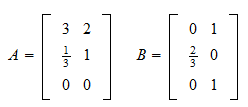Their sum isRemember that column vectors and row vectors are also matrices. As a consequence, they can be summed in the same way, as shown by the following example.

Example Letandbe twocolumn vectors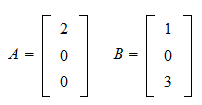Their sum is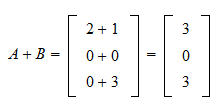## Properties of matrix addition

Matrix addition enjoys properties that are similar to those enjoyed by the more familiar addition of real numbers.

Proposition (commutative property) Matrix addition is commutative, that is,for any matricesandsuch that the above additions are meaningfully defined.

Proof

This is an immediate consequence of the fact that the commutative property applies to sums of scalars, and therefore to the element-by-element sums that are performed when carrying out matrix addition.

Proposition (associative property) Matrix addition is associative, that is,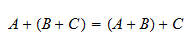for any matrices,andsuch that the above additions are meaningfully defined.

Proof

This is an immediate consequence of the fact that the associative property applies to sums of scalars, and therefore to the element-by-element sums that are performed when carrying out matrix addition.

## Solved exercises

Below you can find some exercises with explained solutions.

### Exercise 1

Letandbematrices defined by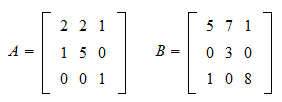Find their sum.

Solution

In order to compute the sum ofand, we need to sum each element ofwith the corresponding element of: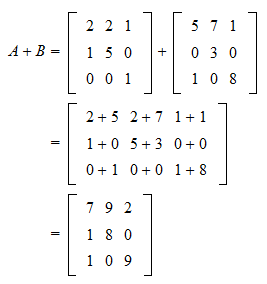### Exercise 2

Letbe the followingmatrix:Define thematrixas follows:Computewhereis the transpose of.

Solution

The transposeis a matrix such that its columns are equal to the rows of:Now, sinceandhave the same dimension, we can compute their sum: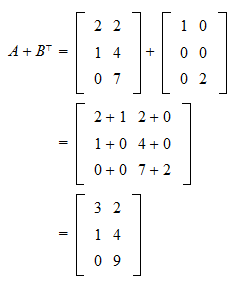### Exercise 3

Letbe amatrix defined byShow that the sum ofand its transpose is a symmetric matrix.

Solution

The transpose ofisThe sum ofandis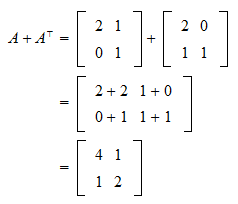Finally,is symmetric if it is equal to its transpose. The latter isThus, the assertion is true.